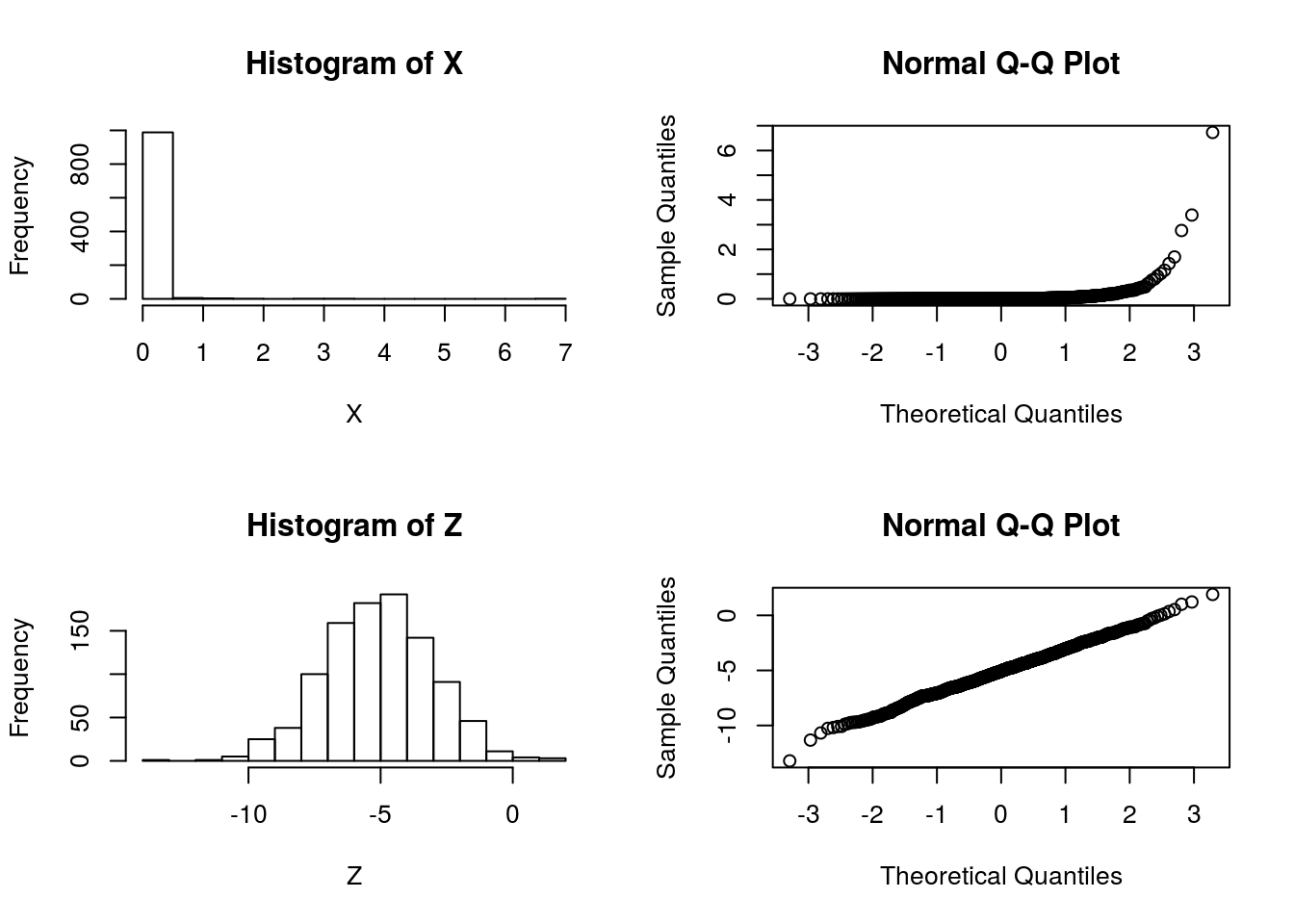Last updated: 2017-03-04

Code version: 5d0fa13282db4a97dc7d62e2d704e88a5afdb824

# Pre-requisites

• Likelihood Ratio.

# Example

Suppose that we are considering whether to model some data $$X$$ as normal or log-normal. In this case we’ll assume the truth is that the data are log normal, which we can simulate as follows:

X = exp(rnorm(1000,-5,2))

We will use $$Z$$ to denote $$\log(X)$$:

Z = log(X)

And let’s check by graphing which looks more normal:

par(mfcol=c(2,2))
hist(X)
hist(Z)
qqnorm(X)
qqnorm(Z)So it is pretty clear that the model $$M_2: \log(X)$$ is normal" is better than the model “$$M_1: X$$ is normal”.

Now consider computing a “log-likelihood” for each model.

To compute a log-likelihood under the model “X is normal” we need to also specify a mean and variance (or standard deviation). We use the sample mean and variance here:

sum(dnorm(X, mean=mean(X), sd=sd(X),log=TRUE))
 -135.3375

Doing the same for $$Z$$ we obtain:

sum(dnorm(Z, mean=mean(Z), sd=sd(Z),log=TRUE))
 -2125.232

Done this way the log-likelihood for $$M_1$$ appears much larger than the log-likelihood for $$M_2$$, contradicting both the graphical evidence and the way the data were simulated.

# The right way

The explanation here is that it does not make sense to compare a likelihood for $$Z$$ with a likelihood for $$X$$ because even though $$Z$$ and $$X$$ are 1-1 mappings of one another ($$Z$$ is determined by $$X$$, and vice versa), they are formally not the same data. That is, it does not make sense to compute $\text{"LLR"} := \log(p(X|M_1)/p(Z|M_2))$.

However, we could compute a log-likelihood ratio for this problem as $\text{LLR} := log(p(X|M_1)/p(X|M_2)).$ Here we are using the fact that the model $$M_2$$ for $$Z$$ actually implies a model for $$X$$: $$Z$$ is normal if and only if $$X$$ is log-normal. So a sensible LLR would be given by:

sum(dnorm(X, mean=mean(X), sd=sd(X),log=TRUE)) - sum(dlnorm(X, meanlog=mean(Z), sdlog=sd(Z),log=TRUE))
 -3080.778

The fact that the LLR is very negative supports the graphical evidence that $$M_2$$ is a much better fitting model (and indeed, as we know – since we simulated the data – $$M_2$$ is the true model).

## Session information

sessionInfo()
R version 3.3.0 (2016-05-03)
Platform: x86_64-apple-darwin13.4.0 (64-bit)
Running under: OS X 10.10.5 (Yosemite)

locale:
 en_NZ.UTF-8/en_NZ.UTF-8/en_NZ.UTF-8/C/en_NZ.UTF-8/en_NZ.UTF-8

attached base packages:
 stats     graphics  grDevices utils     datasets  methods   base

other attached packages:
 tidyr_0.4.1     dplyr_0.5.0     ggplot2_2.1.0   knitr_1.15.1
 MASS_7.3-45     expm_0.999-0    Matrix_1.2-6    viridis_0.3.4
 workflowr_0.3.0 rmarkdown_1.3

loaded via a namespace (and not attached):
 Rcpp_0.12.5      git2r_0.18.0     plyr_1.8.4       tools_3.3.0
 digest_0.6.9     evaluate_0.10    tibble_1.1       gtable_0.2.0
 lattice_0.20-33  shiny_0.13.2     DBI_0.4-1        yaml_2.1.14
 gridExtra_2.2.1  stringr_1.2.0    gtools_3.5.0     rprojroot_1.2
 grid_3.3.0       R6_2.1.2         reshape2_1.4.1   magrittr_1.5
 backports_1.0.5  scales_0.4.0     htmltools_0.3.5  assertthat_0.1
 mime_0.5         colorspace_1.2-6 xtable_1.8-2     httpuv_1.3.3
 labeling_0.3     stringi_1.1.2    munsell_0.4.3   

This site was created with R Markdown